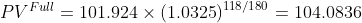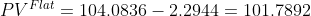Scan QR code or get instant email to install app

Question:

# A 7% U.S. corporate bond is priced for settlement on May 18, 2015. The bond makes semiannual coupon payments on January 20 and July 20 of each year and matures on July 20, 2019. The bond uses the 30/360 day‐count convention for accrued interest. Given that stated annual yield‐ to‐maturity is 6.5%, the flat price of the bond per 100 of par value on May 18, 2015 is closest to:

A \$101.7892.
explanation

1212Given the 30/360 day‐count convention, there are 118 days between the last coupon on January 20, 2015, and the settlement date on May 28, 2015 (10 days between January 20 and January 30, plus 90 days for the full months of February, March, and April, plus 18 days in May). Therefore, the fraction of the coupon period that has gone by equals 118/180. As of the last coupon payment date, there are 4.5 years (and nine semiannual periods) remaining until maturity.
Using stated annual yield‐to‐maturity of 6.5%, or 3.25% per semiannual period, the price of the bond as of the last coupon payment date is calculated as:
N = 9; PMT = –3.50; FV = –100; I/Y = 3.25%; CPT PV; PV = 101.924
The full price on May 18 is calculated as:The accrued interest is calculated as:
AI = 118 / 180 x 3.50 = 2.2944
The flat price is calculated as: